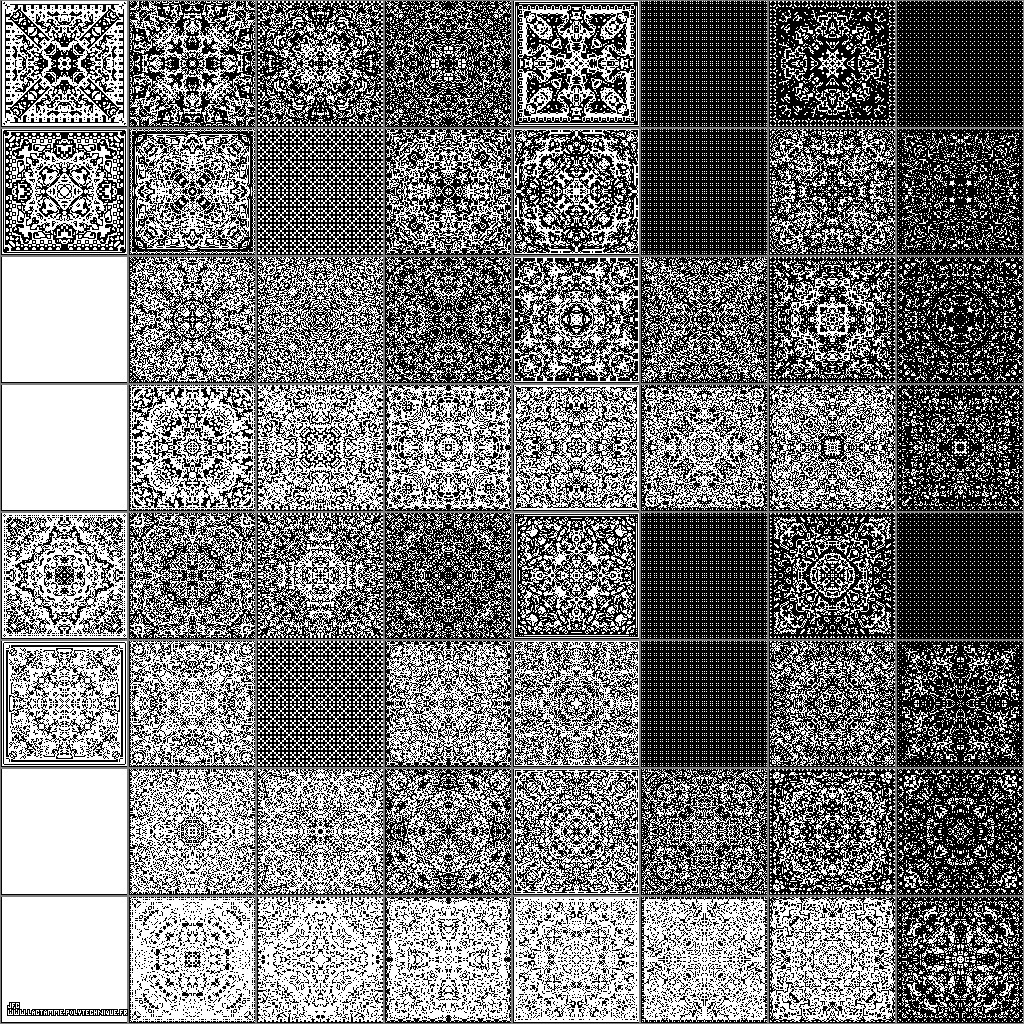64 elementary bidimensional binary cellular automata with 1 white starting central point [64 automates cellulaires binaires bidimensionnels élémentaires avec 1 point central de départ blanc].

An elementary bidimensional binary automaton is a bidimensional set of cells. At time 't', each cell (with coordinate 'x' and 'y') has a value 'CELL(x,y,t)' that equals either 0 (Black) or 1 (White) and has eight neighbours. The points outside the picture are assumed to be Black. The time evolution of this set of cells is defined by means of rules.

For each 3x3 square, the Number of White points NW is computed at time 't'.

This picture displays 8x8 (=64) such elementary bidimensional binary cellular automata. They are computed using the 2^6 (=64) following arbitrary sets of rules:
```                    if (NW(x,y,t)==0) then CELL(x,y,t+1)=B
if (NW(x,y,t)==1) then CELL(x,y,t+1)=W
```
```                    if (NW(x,y,t)==2) then CELL(x,y,t+1)=a (B or W)
if (NW(x,y,t)==3) then CELL(x,y,t+1)=b (B or W)
if (NW(x,y,t)==4) then CELL(x,y,t+1)=c (B or W)
if (NW(x,y,t)==5) then CELL(x,y,t+1)=d (B or W)
if (NW(x,y,t)==6) then CELL(x,y,t+1)=e (B or W)
if (NW(x,y,t)==7) then CELL(x,y,t+1)=f (B or W)
```
```                    if (NW(x,y,t)==8) then CELL(x,y,t+1)=B
if (NW(x,y,t)==9) then CELL(x,y,t+1)=W
```
The 8x8 small images display the 64 following cellular automata:
```                    abcdef    abcdef    abcdef    abcdef    abcdef    abcdef    abcdef    abcdef
```
```                    WWWBBB    BWWBBB    WBWBBB    BBWBBB    WWBBBB    BWBBBB    WBBBBB    BBBBBB
WWWWBB    BWWWBB    WBWWBB    BBWWBB    WWBWBB    BWBWBB    WBBWBB    BBBWBB
WWWBWB    BWWBWB    WBWBWB    BBWBWB    WWBBWB    BWBBWB    WBBBWB    BBBBWB
WWWWWB    BWWWWB    WBWWWB    BBWWWB    WWBWWB    BWBWWB    WBBWWB    BBBWWB
WWWBBW    BWWBBW    WBWBBW    BBWBBW    WWBBBW    BWBBBW    WBBBBW    BBBBBW
WWWWBW    BWWWBW    WBWWBW    BBWWBW    WWBWBW    BWBWBW    WBBWBW    BBBWBW
WWWBWW    BWWBWW    WBWBWW    BBWBWW    WWBBWW    BWBBWW    WBBBWW    BBBBWW
WWWWWW    BWWWWW    WBWWWW    BBWWWW    WWBWWW    BWBWWW    WBBWWW    BBBWWW
```
For each automaton, the number of time steps equals 62.

(CMAP28 WWW site: this page was created on 01/21/2003 and last updated on 04/26/2015 11:18:22 -CEST-)

[See all related pictures (including this one) [Voir toutes les images associées (incluant celle-ci)]]

[Please visit the related NumberTheory picture gallery [Visitez la galerie d'images NumberTheory associée]]
[Go back to AVirtualMachineForExploringSpaceTimeAndBeyond [Retour à AVirtualMachineForExploringSpaceTimeAndBeyond]]
[The Y2K bug [Le bug de l'an 2000]]

[Site Map, Help and Search [Plan du Site, Aide et Recherche]]
[Mail [Courrier]]
[About Pictures and Animations [A Propos des Images et des Animations]]